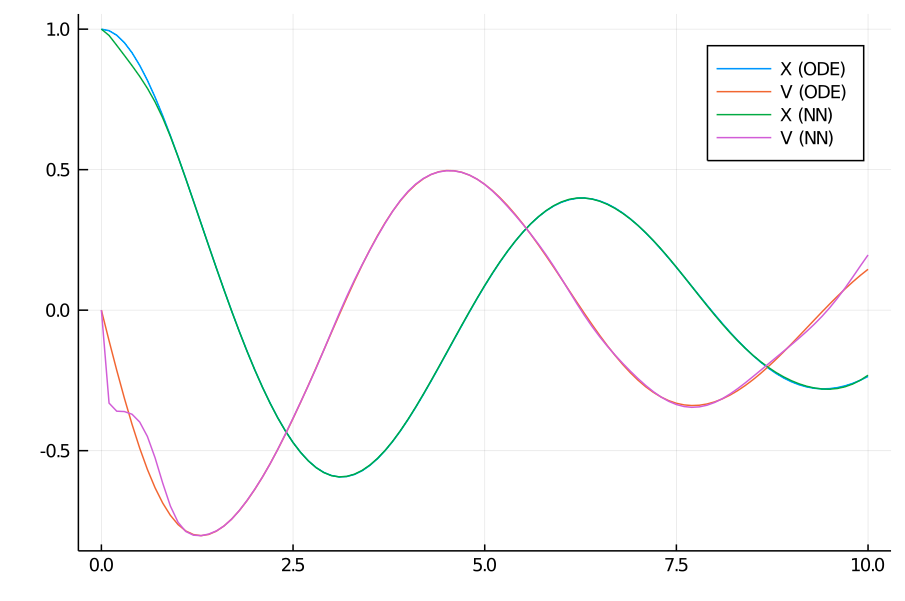# Physics-Informed Machine Learning (PIML) with TensorLayer

In this tutorial, we show how to use the DiffEqFlux TensorLayer to solve problems in Physics Informed Machine Learning.

Let's consider the anharmonic oscillator described by the ODE

$$$ẍ = - kx - αx³ - βẋ -γẋ³.$$$

To obtain the training data, we solve the equation of motion using one of the solvers in DifferentialEquations:

using DiffEqFlux, Optimization, OptimizationFlux, DifferentialEquations, LinearAlgebra
k, α, β, γ = 1, 0.1, 0.2, 0.3
tspan = (0.0,10.0)

function dxdt_train(du,u,p,t)
du = u
du = -k*u - α*u^3 - β*u - γ*u^3
end

u0 = [1.0,0.0]
ts = collect(0.0:0.1:tspan)
prob_train = ODEProblem{true}(dxdt_train,u0,tspan)
data_train = Array(solve(prob_train,Tsit5(),saveat=ts))

Now, we create a TensorLayer that will be able to perform 10th order expansions in a Legendre Basis:

A = [LegendreBasis(10), LegendreBasis(10)]
nn = TensorLayer(A, 1)
(::TensorLayer{Array{DiffEqFlux.TensorProductBasis}, Vector{Float64}, Int64}) (generic function with 2 methods)

and we also instantiate the model we are trying to learn, "informing" the neural about the ∝x and ∝v dependencies in the equation of motion:

f = x -> min(30one(x),x)

function dxdt_pred(du,u,p,t)
du = u
du = -p*u - p*u + f(nn(u,p[3:end]))
end

α = zeros(102)

prob_pred = ODEProblem{true}(dxdt_pred,u0,tspan)

Note that we introduced a "cap" in the neural network term to avoid instabilities in the solution of the ODE. We also initialized the vector of parameters to zero in order to obtain a faster convergence for this particular example.

Finally, we introduce the corresponding loss function:

function predict_adjoint(θ)
x = Array(solve(prob_pred,Tsit5(),p=θ,saveat=ts,
end

loss = sum(norm.(x - data_train))
return loss
end

iter = 0
function callback(θ,l)
global iter
iter += 1
if iter%10 == 0
println(l)
end
return false
end
callback (generic function with 1 method)

and we train the network using two rounds of ADAM:

adtype = Optimization.AutoZygote()
optprob = Optimization.OptimizationProblem(optf, α)
res1 = Optimization.solve(optprob, ADAM(0.05), callback = callback, maxiters = 150)

optprob2 = Optimization.OptimizationProblem(optf, res1.u)
res2 = Optimization.solve(optprob2, ADAM(0.001), callback = callback,maxiters = 150)
opt = res2.u

We plot the results and we obtain a fairly accurate learned model:

using Plots
plot!(ts, data_pred[2,:],label = "V (NN)")